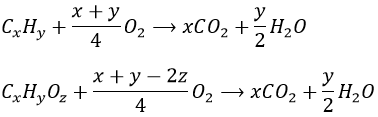# Chapter 1: history of chemistry and chemical reactions

It is often useful to take a look on the history of something to understand it. That is how we will begin our lessons about chemistry. As far as we can go, chemistry started with the discovery of fire, which is basically the combustion of a reactant to obtain heat from it. Later, different metals were discovered, giving the names of the iron, copper and bronze eras.

However, we can’t talk about a scientific method at this moment yet. It is more about evolution. Evolution is a process to adapt best to our environment, most of the time through a trials and errors process. Processes which lead to a better adaptation were repeated while the others were not. Fire for example gave a huge improvement to the life of men for now obvious reasons but yet the process was not deeply understood.

Rationalisation is first seen with the Egyptians (fabrication of glass, beer and coloring), China (porcelain) and then with the Greeks. It is Leucippe and then Democrite who describe the matter as composed of small unbreakable particles, the atomos. Greeks also claimed that the world is composed of 4 main elements: earth, water, air and fire. We could now compare those to the three main phases: solid, liquid, gaz and the energy

The scientific method was developed during the XVI century. The method consists in 3 steps:

1. Observation of a phenomenon: gives quantitative and qualitative information
2. Hypothesis: tries to give possible explanations to the observed phenomenon
3. Experiments: gathers new information on the phenomenon, confirm or not the theories developed in the previous step.

Before that, men described what they saw. From that point, men try to explain what they see through theories.

Stoichiometry and determination of the atomic masses

One of the fathers of the modern chemistry is Lavoisier. The statement he is known for is “Nothing is lost, nothing is created, everything is transformed” which meant that the total mass of the product of a reaction equals the total mass of the reactants. This statement is indeed true except for nuclear reactions during which a part of the mass is converted into energy.

Joseph Proust stated that a chemical compound always contains exactly the same proportion of elements by mass. For example, in pure water, the mass of hydrogen is always 1/9 of the mass of the sample while the oxygen makes up the 8/9 of the mass.

To complete this law, Dalton observed that during a reaction, the masses of the compounds which react together are always in a relation of simple integers. For example oxygen (O) and carbon (C) can react together in several ways

1g of C + 1.33g of O → 2.33g of CO

1g of C + 2.66g of O → 3.66g of CO2

to form carbon monoxide or carbon dioxide. The relationship between the mass of oxygen is 1 to 2. This is the basis of the stoichiometry.  Bertholet protested against that law because one of his experiments gave opposite results. This experiment involved a solid of CuO wherein the ratio between Cu and O is neither constant nor a simple integer. The reason is that solids may have imperfections. Basically, these imperfections can be empty spaces or atoms replaced by others. This is why Bertholet obtained a formula of Cu1-xO instead of CuO.

Dalton established an atomic theory:

• All matter is made of atoms. Atoms are indivisible and indestructible.
• All atoms of a given element are identical in mass and properties. Atoms of different elements are different.
• Compounds are formed by a combination of two or more atoms. There is no formation of new atom (except nuclear reactions).
• A chemical reaction is a rearrangement of atoms.

The mass of each element has been determined. First works were performed by Cannizzaro, basing its experiment on a principle enounced by Avogadro: In normal conditions of temperature and pressure, identical volumes of gas have the same number of particles. Knowing the proportion of carbon there is in different gases, Cannizzaro determined its mass:

 Compound Mass (g) % of carbon Mass of carbon (g) Methane 16 75 12 Ethane 30 80 24 Propane 40 82 36

The mass of C was determined this way. C has a mass of 12 atomic mass units (u). Consecutively, the mass of oxygen (16) has been determined from carbon dioxide (CO2). And so on. Initially, some errors occurred, typically because of elements with an even mass. For example, it was known that 2g of H react with 16g of O to form 18g of water. Considering the simplest relation, H has a mass of 1u (which is correct) but O would have a mass of 8u.

The mole is one of the seven units of the International System of Units (SI Units): kilogram for mass, meter for length, second for time, Kelvin for temperature, ampere for electric current, candela for luminous intensity and mole for the amount of substance. The symbol for mole is mol.

Coming back to Avogadro, one of the most important numbers in chemistry, but almost never used is the Avogadro’s Number NA. As atoms are unbreakable, there are obviously several atoms in 12g of C. A mole expresses the number of atoms of carbon in 12g of carbon.

MC=NA.mC

This relation is true for any element i. Mi is the molar mass of i, i.e. the mass of one mole of the element i. Its units is g/mol (or g mol-1). mi is the mass of one atom of the element i. In the case of the carbon, MC=12g mol-1. mC being a mass, NA unit is mol-1. The value of NA was initially determined by Johann Josef Loschmidt who calculated the number of particles in a given volume of gas. The accuracy of the measure was perfectible and there are now experiments  which give more accurate results than this method.

NA= 6.02214129(27)×1023 mol−1

Ourself and our environment is thus filled by an amazingly large number of atoms that interact together to form matter, air, liquids and most importantly life. The idea that molecules from the living could be crafted was not accepted before the XIX century.  Friedrich Wöhler, a german chemist, can be considered as a pioneer of the organic chemistry. At this time, the hypothesis of vitalism was popular: any compound, to be living, needs a vital force given by God. Humans should not be able to synthesize, without this vital force, any organic compound. Wöhler proved that this theory was wrong by producing urea, accidentally, from inorganic substances. Even if urea is a waste of our body (when we pee), it is an organic compound and it should have been impossible to Wöhler to synthesize it without the intervention of the vital force from a living species. Friedrich Wöhler wanted to produce ammonium cyanate from potassium cyanate (KNCO) and ammonium chloride. However, the target product is unstable and acts only as an intermediary product, decomposing itself into urea.

Stoichiometry

The stoichiometry is the relation between the quantities of reactants and products during a chemical reaction.

A chemical reaction is written by an equation, placing the reactants on the left of an arrow and the products on the right of it. There can be several reactants that react together to form one single product. Each species is separated by a +.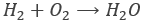In this reaction, hydrogen and oxygen are mixed to produce water (H2O). Hydrogen and oxygen are separated by a + at the left of the arrow because they are the reactants and the water is at the right of the arrow because it is the product of the reaction. Several products can be formed from one or more different reactants.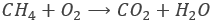In this case, two products are generated by the chemical reaction: water and carbon dioxide. They are also separated by a + and are still at the right of the equation.

Now, the equations are not complete. We have to respect the law of conservation of mass (of Lavoisier): nothing is lost, nothing is created, everything is transformed. The quantities of an atom at the left and at the right of a chemical equation have to be identical. In the first equation, we wrote that one mole of H2 reacts with one mole of O2 to form one mole of H2O. The number of H is equal before and after the reaction (there are 2 of them in H2 and 2 in H2O) but one atom of oxygen would be lost. To obtain the correct equation, we put coefficients, called stoichiometric coefficients, before the species: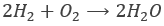It is thus 2 moles of H2 that react with one mole oxygen to produce two moles of water. Here, the quantities of each atom is identical at each side of the arrow. This notation is also correct, as long as the numbers of atom are integers: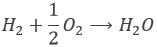The second equation that we wrote was also incorrect: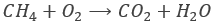The number of carbon C is correct, but the quantities of H and of O changed during the reaction. As there are 4 hydrogen at the left, we will put a coefficient 2 in front of the water to have 4 H at the right of the equation. Now, we have 4 oxygen’s in the products and only 2 in the reactants. To correct the equation, a coefficient 2 is put before the O2. The number of C is still correct and the equation is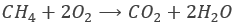There can be some variations to this notation. If a specific solvent is required for a reaction to happen, we indicate it above or under the arrow.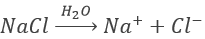This reaction is the dissolution of salt (NaCl, also called table salt, the salt that we add on our food) into water. The elements of the salt are separated into the corresponding ions, i.e. charged species. The positively charged ions are called cations and the negatively charged species are called anions. If we need to heat the solution for the reaction to happen, we will also indicate it near the arrow by a Δ or a ΔT.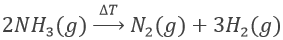A reaction that requires heat to be made is an endothermic reaction. If the reaction generates heat, this reaction is said to be exothermic. Note that in the previous reaction, we indicated the states of the compounds between brackets. The g means that the species are gaseous. A s means that it is a solid, l stands for a liquid and aq stands for an aqueous solution. The heat generated by an exothermic reaction is written just as a product by Q or its exact value in kJ/mol if it is known. Some reactions produce light, also indicated as a product by hν, i.e. a photon of frequency ν.

The last point to talk about is that all the reactions are not complete. A complete reactions means that, if the reactants are put in stoichiometric proportions, all the reactants will be consumed during the reaction to form the products. If one reactant has more than the stoichiometric proportion, it is in excess and there will still be an amount of this reactant after the reaction, corresponding at the excess. During incomplete reactions, called reactions of equilibrium, all the reactants are not consumed even if they are put in stoichiometric proportions. There is an equilibrium between the quantities of reactants and of products of this reaction. Incomplete does not mean that the products are not fully made, but only that a part of them are generated. For example, the acetic acid is a low acid that does not completely dissolve in water.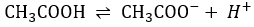As a result, we find the three species in solution: CH3COOH, CH3COO and H+. Some of the reactants formed the products and some did not react. Note that the arrow in the chemical equation is different from the one of complete reactions. It is now two half arrows meaning that the reaction can go in both sides. For equilibrium reactions, we define a constant of equilibrium K such as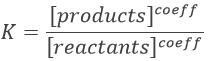The [] means that we consider the concentrations of the species between the [] power their stoichiometric coefficient.

Exercises

1. Equilibrate those equations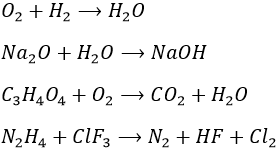2. If we put together 2g of Br2 and 1g of H2, how many moles of HBr can be produced? What is the mass of the excess of reactant?

3. Write the general equation for the combustion of the organic compounds CxHy and CxHyOz

1.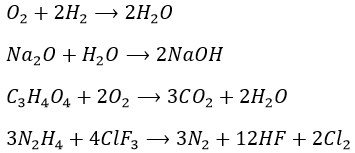2. The reaction consumes 1 mole of each reactant to form 2 moles of HBr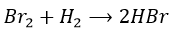As Br has the biggest molar mass, H2 will be in excess.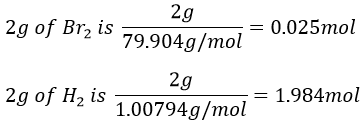As a result, only 0.025mol of H2 is consumed and 0.05mol of HBr is produced by the reaction. The excess of H2 is 1.959molà1.975g of excess.

3. A combustion reaction is the reaction between one reactant and oxygen. From organic molecules, it generates CO2 and water.